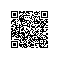# Python机器学习小知识：pandas.apply

pandas.apply函数是Python在机器学习处理数据时常用的一个方法。apply函数会自动遍历每一行DataFrame的数据，最后将所有结果组合成一个Series数据结构并返回。该函数定义如下：
DataFrame.apply(self， func， axis=0， broadcast=None， raw=False， reduce=None， result_type=None， args=()， **kwds)

https://pandas.pydata.org/pandas-docs/stable/reference/api/pandas.DataFrame.apply.html

df = pd.DataFrame([[4, 9]] * 3, columns=['A', 'B'])
df
A B
0 4 9
1 4 9
2 4 9

df.apply(np.sqrt)
A B
0 2.0 3.0
1 2.0 3.0
2 2.0 3.0

df.apply(np.sum, axis=0)
A 12
B 27
dtype: int64

df.apply(np.sum, axis=1)
0 13
1 13
2 13
dtype: int64使用钉钉扫一扫加入圈子
+ 订阅